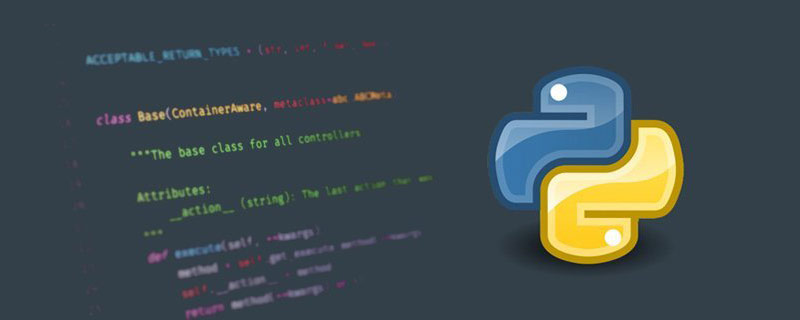# python如何求阶乘_亿码酷站_编程开发技术教程

【亿码酷站-编程开发教程】收集全网优质教程及源码资源！python阶乘的方法：

```a = int(input('please inputer a integer:'))
num = 1
if a < 0:
print('负数没有阶乘！')
elif a == 0:
print('0的阶乘为1！')
else :
for i in range(1,a + 1):
num *= i
print(num)```

```#从functools中调用reduce()函数
from functools import reduce
#使用lambda，匿名函数，迭代
num = reduce(lambda x,y:x*y,range(1,7))
print(num)```

```import math
value = math.factorial(6)
print(value)```

```def num(n):
if n == 0:
return 1
else:
return n * num(n - 1)
print(num(6)```

python如何求阶乘
—–文章转载自PHP中文网如有侵权请联系admin#tyuanma.cn删除

PHP7中“??”和“?:”的区别是什么？

## python print用法是什么_编程技术_亿码酷站

python print用法是什么_编程技术_亿码酷站

## python如何绘制心形_亿码酷站_编程开发技术教程

python如何绘制心形_亿码酷站_编程开发技术教程